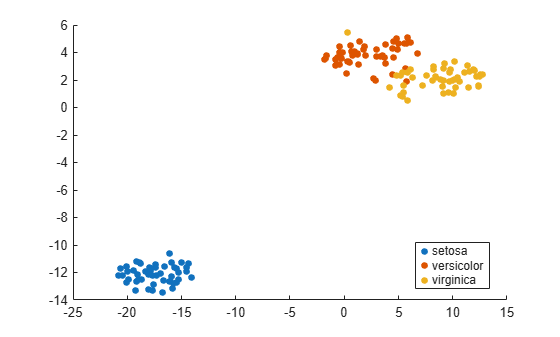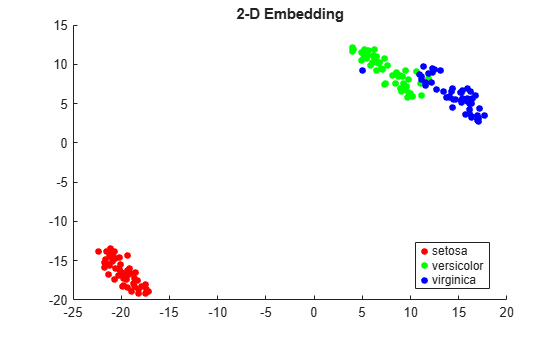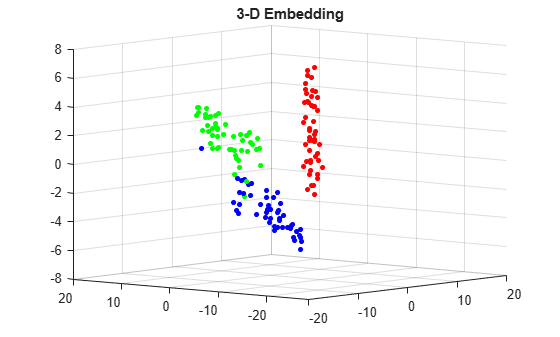# tsne

t-Distributed Stochastic Neighbor Embedding

## Syntax

``Y = tsne(X)``
``Y = tsne(X,Name,Value)``
``````[Y,loss] = tsne(___)``````

## Description

example

````Y = tsne(X)` returns a matrix of two-dimensional embeddings of the high-dimensional rows of `X`.```

example

````Y = tsne(X,Name,Value)` modifies the embeddings using options specified by one or more name-value pair arguments.```

example

``````[Y,loss] = tsne(___)```, for any input arguments, also returns the Kullback-Leibler divergence between the joint distributions that model the data `X` and the embedding `Y`.```

## Examples

collapse all

The Fisher iris data set has four-dimensional measurements of irises, and corresponding classification into species. Visualize this data by reducing the dimension using `tsne`.

```load fisheriris rng default % for reproducibility Y = tsne(meas); gscatter(Y(:,1),Y(:,2),species)```Use various distance metrics to try to obtain a better separation between species in the Fisher iris data.

```load fisheriris rng('default') % for reproducibility Y = tsne(meas,'Algorithm','exact','Distance','mahalanobis'); subplot(2,2,1) gscatter(Y(:,1),Y(:,2),species) title('Mahalanobis') rng('default') % for fair comparison Y = tsne(meas,'Algorithm','exact','Distance','cosine'); subplot(2,2,2) gscatter(Y(:,1),Y(:,2),species) title('Cosine') rng('default') % for fair comparison Y = tsne(meas,'Algorithm','exact','Distance','chebychev'); subplot(2,2,3) gscatter(Y(:,1),Y(:,2),species) title('Chebychev') rng('default') % for fair comparison Y = tsne(meas,'Algorithm','exact','Distance','euclidean'); subplot(2,2,4) gscatter(Y(:,1),Y(:,2),species) title('Euclidean')```In this case, the cosine, Chebychev, and Euclidean distance metrics give reasonably good separation of clusters. But the Mahalanobis distance metric does not give a good separation.

`tsne` removes input data rows that contain any `NaN` entries. Therefore, you must remove any such rows from your classification data before plotting.

For example, change a few random entries in the Fisher iris data to `NaN`.

```load fisheriris rng default % for reproducibility meas(rand(size(meas)) < 0.05) = NaN;```

Embed the four-dimensional data into two dimensions using `tsne`.

`Y = tsne(meas,'Algorithm','exact');`
```Warning: Rows with NaN missing values in X or 'InitialY' values are removed. ```

Determine how many rows were eliminated from the embedding.

`length(species)-length(Y)`
```ans = 22 ```

Prepare to plot the result by locating the rows of `meas` that have no `NaN` values.

`goodrows = not(any(isnan(meas),2));`

Plot the results using only the rows of `species` that correspond to rows of `meas` with no `NaN` values.

`gscatter(Y(:,1),Y(:,2),species(goodrows))`Find both 2-D and 3-D embeddings of the Fisher iris data, and compare the loss for each embedding. It is likely that the loss is lower for a 3-D embedding, because this embedding has more freedom to match the original data.

```load fisheriris rng default % for reproducibility [Y,loss] = tsne(meas,'Algorithm','exact'); rng default % for fair comparison [Y2,loss2] = tsne(meas,'Algorithm','exact','NumDimensions',3); fprintf('2-D embedding has loss %g, and 3-D embedding has loss %g.\n',loss,loss2)```
```2-D embedding has loss 0.12929, and 3-D embedding has loss 0.0992412. ```

As expected, the 3-D embedding has lower loss.

View the embeddings. Use RGB colors `[1 0 0]`, `[0 1 0]`, and `[0 0 1]`.

For the 3-D plot, convert the species to numeric values using the `categorical` command, then convert the numeric values to RGB colors using the `sparse` function as follows. If `v` is a vector of positive integers 1, 2, or 3, corresponding to the species data, then the command

`sparse(1:numel(v),v,ones(size(v)))`

is a sparse matrix whose rows are the RGB colors of the species.

```gscatter(Y(:,1),Y(:,2),species,eye(3)) title('2-D Embedding')``````figure v = double(categorical(species)); c = full(sparse(1:numel(v),v,ones(size(v)),numel(v),3)); scatter3(Y2(:,1),Y2(:,2),Y2(:,3),15,c,'filled') title('3-D Embedding') view(-50,8)```## Input Arguments

collapse all

Data points, specified as an `n`-by-`m` matrix, where each row is one `m`-dimensional point.

`tsne` removes rows of `X` that contain any `NaN` values before creating an embedding. See Plot Results with NaN Input Data.

Data Types: `single` | `double`

### Name-Value Arguments

Specify optional pairs of arguments as `Name1=Value1,...,NameN=ValueN`, where `Name` is the argument name and `Value` is the corresponding value. Name-value arguments must appear after other arguments, but the order of the pairs does not matter.

Before R2021a, use commas to separate each name and value, and enclose `Name` in quotes.

Example: ```Y = tsne(X,'Algorithm','Exact','NumPCAComponents',50)```

Algorithm Control

collapse all

`tsne` algorithm, specified as `'barneshut'` or `'exact'`. The `'exact'` algorithm optimizes the Kullback-Leibler divergence of distributions between the original space and the embedded space. The `'barneshut'` algorithm performs an approximate optimization that is faster and uses less memory when the number of data rows is large.

Note

For the `'barneshut'` algorithm, `tsne` uses `knnsearch` to find the nearest neighbors.

Example: `'exact'`

Size of the Gram matrix in megabytes, specified as a positive scalar or `"maximal"`. The `tsne` function can use `CacheSize` only when the `Distance` name-value argument begins with `fast`.

If you set `CacheSize` to `"maximal"`, `tsne` tries to allocate enough memory for an entire intermediate matrix whose size is `M`-by-`M`, where `M` is the number of rows of the input data `X`. The cache size does not have to be large enough for an entire intermediate matrix, but must be at least large enough to hold an `M`-by-1 vector. Otherwise, `tsne` uses the standard algorithm for computing Euclidean distances.

If the value of the `Distance` argument begins with `fast`, and the value of `CacheSize` is too large or `"maximal"`, `tsne` might try to allocate a Gram matrix that exceeds the available memory. In this case, MATLAB® issues an error.

Example: `CacheSize="maximal"`

Data Types: `double` | `char` | `string`

Distance metric, specified as one of the following:

• `'euclidean'` — Euclidean distance.

• `'seuclidean'` — Standardized Euclidean distance. Each coordinate difference between the rows in `X` and the query matrix is scaled by dividing by the corresponding element of the standard deviation computed from `S = std(X,'omitnan')`.

• `'fasteuclidean'` — Euclidean distance computed by using an alternative algorithm that saves time when the number of predictors is at least 10. In some cases, this faster algorithm can reduce accuracy. Algorithms starting with `'fast'` do not support sparse data. For details, see Algorithms.

• `'fastseuclidean'` — Standardized Euclidean distance computed by using an alternative algorithm that saves time when the number of predictors is at least 10. In some cases, this faster algorithm can reduce accuracy. Algorithms starting with `'fast'` do not support sparse data. For details, see Algorithms.

• `'cityblock'` — City block distance.

• `'chebychev'` — Chebychev distance, which is the maximum coordinate difference.

• `'minkowski'` — Minkowski distance with exponent 2. This distance is the same as the Euclidean distance.

• `'mahalanobis'` — Mahalanobis distance, computed using the positive definite covariance matrix `cov(X,'omitrows')`.

• `'cosine'` — One minus the cosine of the included angle between observations (treated as vectors).

• `'correlation'` — One minus the sample linear correlation between observations (treated as sequences of values).

• `'spearman'` — One minus the sample Spearman's rank correlation between observations (treated as sequences of values).

• `'hamming'` — Hamming distance, which is the percentage of coordinates that differ.

• `'jaccard'` — One minus the Jaccard coefficient, which is the percentage of nonzero coordinates that differ.

• Custom distance function — A distance function specified using `@` (for example, `@distfun`). For details, see More About.

In all cases, `tsne` uses squared pairwise distances to calculate the Gaussian kernel in the joint distribution of `X`.

Example: `'mahalanobis'`

Data Types: `char` | `string` | `function_handle`

Size of natural clusters in data, specified as a scalar value `1` or greater.

A large exaggeration makes `tsne` learn larger joint probabilities of `Y` and creates relatively more space between clusters in `Y`. `tsne` uses exaggeration in the first 99 optimization iterations.

If the value of Kullback-Leibler divergence increases in the early stage of the optimization, try reducing the exaggeration. See tsne Settings.

Example: `10`

Data Types: `single` | `double`

Dimension of the output `Y`, specified as a positive integer. Generally, set `NumDimensions` to `2` or `3`.

Example: `3`

Data Types: `single` | `double`

PCA dimension reduction, specified as a nonnegative integer. Before `tsne` embeds the high-dimensional data, it first reduces the dimensionality of the data to `NumPCAComponents` using the `pca` function. When `NumPCAComponents` is `0`, `tsne` does not use PCA.

Example: `50`

Data Types: `single` | `double`

Effective number of local neighbors of each point, specified as a positive scalar. See t-SNE Algorithm.

Larger perplexity causes `tsne` to use more points as nearest neighbors. Use a larger value of `Perplexity` for a large dataset. Typical `Perplexity` values are from `5` to `50`. In the Barnes-Hut algorithm, `tsne` uses `min(3*Perplexity,N-1)` as the number of nearest neighbors. See tsne Settings.

Example: `10`

Data Types: `single` | `double`

Flag to normalize input data, specified as `false` or `true`. When the value is `true`, `tsne` centers and scales each column of `X` by first subtracting its mean, and then dividing by its standard deviation.

When features in `X` are on different scales, set `'Standardize'` to `true`. The learning process is based on nearest neighbors, so features with large scales can override the contribution of features with small scales.

Example: `true`

Data Types: `logical`

Optimization Control

collapse all

Initial embedded points, specified as an `n`-by-`NumDimensions` real matrix, where `n` is the number of rows of `X`. The `tsne` optimization algorithm uses these points as initial values.

Data Types: `single` | `double`

Learning rate for optimization process, specified as a positive scalar. Typically, set values from `100` through `1000`.

When `LearnRate` is too small, `tsne` can converge to a poor local minimum. When `LearnRate` is too large, the optimization can initially have the Kullback-Leibler divergence increase rather than decrease. See tsne Settings.

Example: `1000`

Data Types: `single` | `double`

Iterative display frequency, specified as a positive integer. When the `Verbose` name-value pair is not `0`, `tsne` returns iterative display after every `NumPrint` iterations. If the `Options` name-value pair contains a nonempty `'OutputFcn'` entry, then output functions run after every `NumPrint` iterations.

Example: `20`

Data Types: `single` | `double`

Optimization options, specified as a structure containing the fields `'MaxIter'`, `'OutputFcn'`, and `'TolFun'`. Create `'Options'` using `statset` or `struct`.

• `'MaxIter'` — Positive integer specifying the maximum number of optimization iterations. Default: `1000`.

• `'OutputFcn'` — Function handle or cell array of function handles specifying one or more functions to call after every `NumPrint` optimization iterations. For syntax details, see t-SNE Output Function. Default: `[]`.

• `'TolFun'` — Stopping criterion for the optimization. The optimization exits when the norm of the gradient of the Kullback-Leibler divergence is less than `'TolFun'`. Default: `1e-10`.

Example: `options = statset('MaxIter',500)`

Data Types: `struct`

Barnes-Hut tradeoff parameter, specified as a scalar from 0 through 1. Higher values give a faster but less accurate optimization. Applies only when `Algorithm` is `'barneshut'`.

Example: `0.1`

Data Types: `single` | `double`

Iterative display, specified as `0`, `1`, or `2`. When `Verbose` is not `0`, `tsne` prints a summary table of the Kullback-Leibler divergence and the norm of its gradient every `NumPrint` iterations.

When `Verbose` is `2`, `tsne` also prints the variances of Gaussian kernels. `tsne` uses these kernels in its computation of the joint probability of `X`. If you see a large difference in the scales of the minimum and maximum variances, you can sometimes get more suitable results by rescaling `X`.

Example: `2`

Data Types: `single` | `double`

## Output Arguments

collapse all

Embedded points, returned as an `n`-by-`NumDimensions` matrix. Each row represents one embedded point. `n` is the number of rows of data `X` that do not contain any `NaN` entries. See Plot Results with NaN Input Data.

Kullback-Leibler divergence between modeled input and output distributions, returned as a nonnegative scalar. For details, see t-SNE Algorithm.

collapse all

### Custom Distance Function

The syntax of a custom distance function is as follows.

`function D2 = distfun(ZI,ZJ)`

`tsne` passes `ZI` and `ZJ` to your function, and your function computes the distance.

• `ZI` is a 1-by-n vector containing a single row from `X` or `Y`.

• `ZJ` is an m-by-n matrix containing multiple rows of `X` or `Y`.

Your function returns `D2`, which is an m-by-1 vector of distances. The jth element of `D2` is the distance between the observations `ZI` and `ZJ(j,:)`.

Tip

If your data are not sparse, then usually the built-in distance functions are faster than a function handle.

## Algorithms

collapse all

`tsne` constructs a set of embedded points in a low-dimensional space whose relative similarities mimic those of the original high-dimensional points. The embedded points show the clustering in the original data.

Roughly, the algorithm models the original points as coming from a Gaussian distribution, and the embedded points as coming from a Student’s t distribution. The algorithm tries to minimize the Kullback-Leibler divergence between these two distributions by moving the embedded points.

For details, see t-SNE.

### Fast Euclidean Distance Algorithm

The values of the `Distance` argument that begin `fast` (such as `'fasteuclidean'` and `'fastseuclidean'`) calculate Euclidean distances using an algorithm that uses extra memory to save computational time. This algorithm is named "Euclidean Distance Matrix Trick" in Albanie  and elsewhere. Internal testing shows that this algorithm saves time when the number of predictors is at least 10. Algorithms starting with `'fast'` do not support sparse data.

To find the matrix D of distances between all the points xi and xj, where each xi has n variables, the algorithm computes distance using the final line in the following equations:

`$\begin{array}{c}{D}_{i,j}^{2}=‖{x}_{i}-{x}_{j}{‖}^{2}\\ ={\left(}^{{x}_{i}}\left({x}_{i}-{x}_{j}\right)\\ =‖{x}_{i}{‖}^{2}-2{x}_{i}^{T}{x}_{j}+‖{x}_{j}{‖}^{2}.\end{array}$`

The matrix ${x}_{i}^{T}{x}_{j}$ in the last line of the equations is called the Gram matrix. Computing the set of squared distances is faster, but slightly less numerically stable, when you compute and use the Gram matrix instead of computing the squared distances by squaring and summing. For a discussion, see Albanie .

To store the Gram matrix, the software uses a cache with the default size of `1e3` megabytes. You can set the cache size using the `CacheSize` name-value argument. If the value of `CacheSize` is too large or `"maximal"`, `tsne` might try to allocate a Gram matrix that exceeds the available memory. In this case, MATLAB issues an error.

 Albanie, Samuel. Euclidean Distance Matrix Trick. June, 2019. Available at https://www.robots.ox.ac.uk/%7Ealbanie/notes/Euclidean_distance_trick.pdf.

## Version History

Introduced in R2017a

expand all